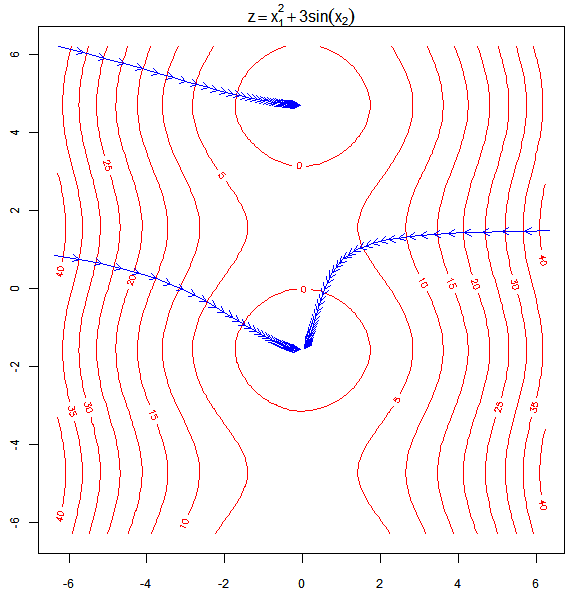# Process of Minimization by Gradient Descent (2D)

### Yihui Xie 2007-09-06

This demo was written by me about three months ago when I was illustrating the algorithm of Gradient Descent in the class of “Data Mining & Machine Learning”. I like to combine iterations (or loopings) with animated pictures, because it’s simple and heuristic, and of course, it’s easy in R: just use `Sys.sleep()` to control the time of steps of your demonstration and some low-level graphics functions such as `lines()`, `points()`, `rect()`, `polygon()` and `segments()`, etc to illustrate the process of your algorithm. To understand the figure below, you need to be clear about what’s `contour plot`.Below is the code for the above example, and it has been included in the animation package as the `grad.desc()` function:

``````op = par(
pty = "s", mar = c(2, 1, 2, 0), cex.axis = 0.75, cex.main = 0.85
)
x2 = x1 = seq(-2 * pi, 2 * pi, 0.1)
f = function(x, y) x^2 + 3 * sin(y)
contour(x1, x2, outer(x1, x2, f), col = "red", main = "")
mtext(side = 3, expression(z == x^2 + 3 * sin(x)))
for (i in 1:3) {
x = unlist(locator(1))
s = 0.05
newx = x - s * c(2 * x, 3 * cos(x))
eps = 0.001
gap = abs(f(newx, newx) - f(x, x))
while (gap > eps) {
arrows(x, x, newx, newx, length = 0.075, col = "blue")
x = newx
newx = x - s * c(2 * x, 3 * cos(x))
gap = abs(f(newx, newx) - f(x, x))
Sys.sleep(gap/3)
}
}
par(op)
``````